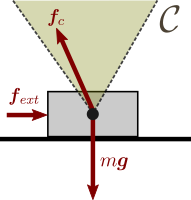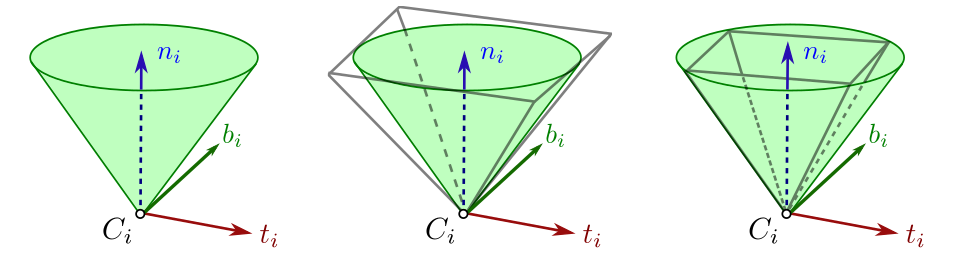# Friction conesConsider the 2D example depicted in figure to the right. A 2D mass is in contact with a single surface. This contact will remain fixed as long as the contact force $$\bff^c = m \bfg - \bff^{\mathit{ext}}$$ lies within the Coulomb friction cone $$\calC$$. As soon as $$\bff^c$$ exits $$\calC$$, the contact switches to the sliding mode. The property $$\bff^c \in \calC$$ is called the contact-stability condition for this contact mode: as long as it is fulfilled, the contact remains fixed. Deriving contact-stability conditions for multibody systems (such as ZMP support areas) is of key interest as robots are commonly controlled using only a small number of contact modes.

## Coulomb friction

Consider the set of points $$\{C_i\}$$ where the robot contacts its environment. Assuming that the environment surface is smooth enough, one can consider its unit normal $$\bfn_i$$ at $$C_i$$, pointing from the environment to the robot. Let $$\bff^c_i$$ denote the contact force exerted at $$C_i$$ by the environment onto the robot:

• the normal component $$\bff^n_i \defeq (\bfn_i \cdot \bff^c_i) \bfn_i$$ is the normal force, and
• the tangential component $$\bff^t_i \defeq \bff^c_i - (\bfn_i \cdot \bff^c_i) \bfn_i$$ is the friction force at $$C_i$$.

A point contact remains in the fixed contact mode while its contact force $$\bff^c_i$$ lies inside the friction cone directed by $$\bfn_i$$:

\begin{equation} (\bff^c_i \cdot \bfn_i) \ > \ 0, \quad \textrm{and} \quad \left\| \bff^t_i \right\|_2 \ \leq \ \mu_i (\bff^{c}_i \cdot \bfn_i), \end{equation}

where $$\mu_i$$ is the static friction coefficient at contact $$C_i$$. The Euclidean norm $$\| \cdot \|_2$$ in this definition represents friction cones with circular sections, which models the isotropy of friction. Although more realistic, this model presents some computational challenges down the control pipeline. A common practice is to consider its linear approximation:

## Linearized friction cones

A point contact remains in the fixed contact mode while its contact force $$\bff^c_i$$ lies inside the linearized friction cone directed by $$\bfn_i$$:

\begin{equation} \bff^c_i \cdot \bfn_i \ > \ 0, \quad \textrm{and} \quad \frac{\bff^t_i}{\tilde{\mu}_i (\bff^{c}_i \cdot \bfn_i)} \ \in \ \calP_n, \end{equation}

where $$\calP_n$$ is the regular $$n$$-sided polygon inscribed in the 2D unit circle.

This approximation can be made as close as desired to the original model by increasing the number of edges $$n$$ of the section polygon $$\calP_n$$. The equation above provides a set of linear inequality constraints. For example, the four-sided friction pyramid obtained for $$n=4$$ can be written:

\begin{eqnarray} \bff^c_i \cdot \bfn_i & > & 0 \\ | \bff^{c}_i \cdot \bft_i | & \leq & \tilde{\mu}_i (\bff^{c}_i \cdot \bfn_i), \\ | \bff^{c}_i \cdot \bfb_i | & \leq & \tilde{\mu}_i (\bff^{c}_i \cdot \bfn_i), \end{eqnarray}

with $$(\bft_i, \bfb_i)$$ any basis of the tangential contact plane such that $$(\bft_i, \bfb_i, \bfn_i)$$ is a direct frame. For $$\tilde{\mu}_i = \mu_i$$, the linearized Coulomb cone is an outer approximation of the circular one, while it is an inner approximation for $$\tilde{\mu}_i = \mu_i / \sqrt{2}$$:Coulomb friction cone (left) with outer (middle) and inner (right) linear approximations.

## To go further

Individual friction cones can be combined into wrench friction cones using polyhedral geometry. We can for instance apply the double description method to compute centroidal wrench cones of articulated robots.

Pages of this website are under the CC-BY 4.0 license.